# Integral Geometry

In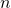-dimensional Euclidean space, the Minkowski functionals can be defined by the Steiner formula [bibcite key=schneider2008stochastic]. Given a convex body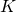, i.e., a compact, convex subset of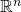, the parallel body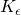at a distanceis the set of all points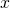for which there is a point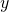insuch that the distance betweenandis smaller or equal to. The volumeof this parallel bodycan be expressed by a polynomial of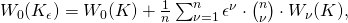where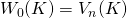and the coefficients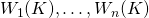depend onbut not on. The so-called Minkowski functionals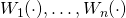can be generalized to finite unions of convex bodies, so that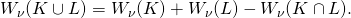This is the additivity property [bibcite key=schneider2008stochastic]. By this choice of normalization, the zeroth order Minkowski functional of an-dimensional unit ball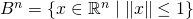is equal to its volume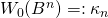, and the higher-order Minkowski functionals are equal to its surface area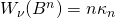(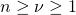). In the mathematical literature the intrinsic volumes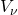are more commonly used than the Minkowski functionals, but only differ by a proportionality constant and the order of the indices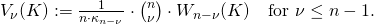The Minkowski tensors of a convex bodyin the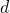-dimensional Euclidean space can be defined using the so-called support measures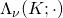, see [bibcite key=schneider2008stochastic], which can in turn be defined by a local Steiner formula. Their total mass yields the intrinsic volumes, that is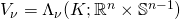. Like the scalar functionals, the tensors can be generalized to non-convex bodies by using their additivity, see [bibcite key=schneider2008stochastic]. For consistency with the Minkowski functionals, we use for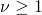a different normalization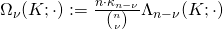. The Minkowski tensors are then defined as [bibcite key=schneider2008stochastic,mcmullen1997isometry]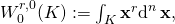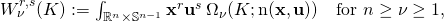where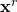or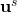are symmetric tensor products, and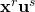is the symmetric tensor product of the tensorsand.

(The presentation follows reference [bibcite key=klatt2017cell].)